# Mean State

Period Mean (original grids) [%]
Model Period Mean (intersection) [%]
Model Period Mean (complement) [%]
Benchmark Period Mean (intersection) [%]
Benchmark Period Mean (complement) [%]
Bias [%]
RMSE [%]
Phase Shift [months]
Bias Score 
RMSE Score 
Seasonal Cycle Score 
Spatial Distribution Score 
Interannual Variability Score 
Overall Score 
Benchmark [-] 77.9
CLM5PHSOFF [-] 70.0 70.1 0.00 77.8 80.3 -7.83 13.6 1.96 0.34 0.24 0.72 1.0 0.50 0.51
CLM5PHSON [-] 68.7 68.9 0.00 77.8 80.3 -8.28 14.1 1.93 0.34 0.23 0.72 1.0 0.48 0.50
Period Mean (original grids) [%]
Model Period Mean (intersection) [%]
Model Period Mean (complement) [%]
Benchmark Period Mean (intersection) [%]
Benchmark Period Mean (complement) [%]
Bias [%]
RMSE [%]
Phase Shift [months]
Bias Score 
RMSE Score 
Seasonal Cycle Score 
Spatial Distribution Score 
Interannual Variability Score 
Overall Score 
Benchmark [-] 53.6
CLM5PHSOFF [-] 51.4 50.8 0.00 53.2 77.5 -2.44 11.8 0.555 0.73 0.59 0.93 0.97 0.84 0.77
CLM5PHSON [-] 51.0 50.5 0.00 53.2 77.5 -2.52 11.8 0.548 0.73 0.59 0.93 0.97 0.84 0.78
Period Mean (original grids) [%]
Model Period Mean (intersection) [%]
Model Period Mean (complement) [%]
Benchmark Period Mean (intersection) [%]
Benchmark Period Mean (complement) [%]
Bias [%]
RMSE [%]
Phase Shift [months]
Bias Score 
RMSE Score 
Seasonal Cycle Score 
Spatial Distribution Score 
Interannual Variability Score 
Overall Score 
Benchmark [-] 64.2
CLM5PHSOFF [-] 62.5 62.3 0.00 63.5 77.8 -1.21 11.3 1.10 0.60 0.44 0.85 0.97 0.76 0.68
CLM5PHSON [-] 61.1 61.1 0.00 63.5 77.8 -1.12 11.5 1.17 0.60 0.44 0.84 0.97 0.75 0.67
Period Mean (original grids) [%]
Model Period Mean (intersection) [%]
Model Period Mean (complement) [%]
Benchmark Period Mean (intersection) [%]
Benchmark Period Mean (complement) [%]
Bias [%]
RMSE [%]
Phase Shift [months]
Bias Score 
RMSE Score 
Seasonal Cycle Score 
Spatial Distribution Score 
Interannual Variability Score 
Overall Score 
Benchmark [-] 77.6
CLM5PHSOFF [-] 93.6 88.9 0.00 77.1 83.4 11.8 17.9 2.90 0.11 0.076 0.53 0.71 0.57 0.35
CLM5PHSON [-] 88.5 88.5 0.00 77.1 83.4 11.6 17.7 2.76 0.11 0.079 0.55 0.73 0.55 0.35
Period Mean (original grids) [%]
Model Period Mean (intersection) [%]
Model Period Mean (complement) [%]
Benchmark Period Mean (intersection) [%]
Benchmark Period Mean (complement) [%]
Bias [%]
RMSE [%]
Phase Shift [months]
Bias Score 
RMSE Score 
Seasonal Cycle Score 
Spatial Distribution Score 
Interannual Variability Score 
Overall Score 
Benchmark [-] 84.2
CLM5PHSOFF [-] 84.8 84.8 0.00 85.0 81.2 -0.214 5.07 1.44 0.60 0.25 0.79 0.99 0.54 0.57
CLM5PHSON [-] 85.2 85.2 0.00 85.0 81.2 -0.688 5.23 1.42 0.59 0.24 0.80 1.0 0.52 0.56
Period Mean (original grids) [%]
Model Period Mean (intersection) [%]
Model Period Mean (complement) [%]
Benchmark Period Mean (intersection) [%]
Benchmark Period Mean (complement) [%]
Bias [%]
RMSE [%]
Phase Shift [months]
Bias Score 
RMSE Score 
Seasonal Cycle Score 
Spatial Distribution Score 
Interannual Variability Score 
Overall Score 
Benchmark [-] 72.3
CLM5PHSOFF [-] 82.8 82.8 0.00 72.2 78.9 10.6 18.6 2.07 0.32 0.19 0.69 0.97 0.58 0.49
CLM5PHSON [-] 82.6 82.6 0.00 72.2 78.9 10.4 17.9 2.16 0.32 0.20 0.68 0.97 0.57 0.49
Period Mean (original grids) [%]
Model Period Mean (intersection) [%]
Model Period Mean (complement) [%]
Benchmark Period Mean (intersection) [%]
Benchmark Period Mean (complement) [%]
Bias [%]
RMSE [%]
Phase Shift [months]
Bias Score 
RMSE Score 
Seasonal Cycle Score 
Spatial Distribution Score 
Interannual Variability Score 
Overall Score 
Benchmark [-] 61.3
CLM5PHSOFF [-] 65.7 65.9 0.00 61.0 71.3 4.95 16.2 2.01 0.54 0.32 0.70 0.99 0.76 0.61
CLM5PHSON [-] 65.2 65.4 0.00 61.0 71.3 5.02 16.2 1.97 0.54 0.32 0.70 0.99 0.76 0.61
Period Mean (original grids) [%]
Model Period Mean (intersection) [%]
Model Period Mean (complement) [%]
Benchmark Period Mean (intersection) [%]
Benchmark Period Mean (complement) [%]
Bias [%]
RMSE [%]
Phase Shift [months]
Bias Score 
RMSE Score 
Seasonal Cycle Score 
Spatial Distribution Score 
Interannual Variability Score 
Overall Score 
Benchmark [-] 69.0
CLM5PHSOFF [-] 64.4 64.0 0.00 68.3 79.0 -4.35 11.7 0.928 0.60 0.39 0.87 0.94 0.66 0.64
CLM5PHSON [-] 62.1 61.8 0.00 68.3 79.0 -4.10 11.5 0.920 0.61 0.39 0.87 0.94 0.65 0.64
Period Mean (original grids) [%]
Model Period Mean (intersection) [%]
Model Period Mean (complement) [%]
Benchmark Period Mean (intersection) [%]
Benchmark Period Mean (complement) [%]
Bias [%]
RMSE [%]
Phase Shift [months]
Bias Score 
RMSE Score 
Seasonal Cycle Score 
Spatial Distribution Score 
Interannual Variability Score 
Overall Score 
Benchmark [-] 78.8
CLM5PHSOFF [-] 89.0 89.0 0.00 78.5 84.9 10.4 16.4 2.77 0.15 0.098 0.56 0.82 0.52 0.38
CLM5PHSON [-] 88.9 88.9 0.00 78.5 84.9 10.5 16.2 3.05 0.15 0.10 0.50 0.83 0.52 0.37
Period Mean (original grids) [%]
Model Period Mean (intersection) [%]
Model Period Mean (complement) [%]
Benchmark Period Mean (intersection) [%]
Benchmark Period Mean (complement) [%]
Bias [%]
RMSE [%]
Phase Shift [months]
Bias Score 
RMSE Score 
Seasonal Cycle Score 
Spatial Distribution Score 
Interannual Variability Score 
Overall Score 
Benchmark [-] 53.1
CLM5PHSOFF [-] 52.7 52.6 0.00 51.3 74.6 1.26 6.89 0.482 0.74 0.52 0.92 1.0 0.81 0.75
CLM5PHSON [-] 50.0 50.0 0.00 51.3 74.6 1.27 6.95 0.506 0.74 0.52 0.91 1.0 0.80 0.75
Period Mean (original grids) [%]
Model Period Mean (intersection) [%]
Model Period Mean (complement) [%]
Benchmark Period Mean (intersection) [%]
Benchmark Period Mean (complement) [%]
Bias [%]
RMSE [%]
Phase Shift [months]
Bias Score 
RMSE Score 
Seasonal Cycle Score 
Spatial Distribution Score 
Interannual Variability Score 
Overall Score 
Benchmark [-] 76.7
CLM5PHSOFF [-] 77.1 77.1 0.00 76.6 78.3 0.511 9.37 0.884 0.60 0.31 0.88 0.76 0.63 0.58
CLM5PHSON [-] 77.5 77.5 0.00 76.6 78.3 0.920 9.32 0.889 0.61 0.31 0.88 0.78 0.62 0.58
Period Mean (original grids) [%]
Model Period Mean (intersection) [%]
Model Period Mean (complement) [%]
Benchmark Period Mean (intersection) [%]
Benchmark Period Mean (complement) [%]
Bias [%]
RMSE [%]
Phase Shift [months]
Bias Score 
RMSE Score 
Seasonal Cycle Score 
Spatial Distribution Score 
Interannual Variability Score 
Overall Score 
Benchmark [-] 74.9
CLM5PHSOFF [-] 68.2 70.7 0.00 65.3 79.6 5.44 14.1 1.54 0.41 0.30 0.76 0.95 0.65 0.56
CLM5PHSON [-] 69.5 69.5 0.00 65.3 79.6 5.43 14.1 1.53 0.41 0.30 0.76 0.95 0.64 0.56
Period Mean (original grids) [%]
Model Period Mean (intersection) [%]
Model Period Mean (complement) [%]
Benchmark Period Mean (intersection) [%]
Benchmark Period Mean (complement) [%]
Bias [%]
RMSE [%]
Phase Shift [months]
Bias Score 
RMSE Score 
Seasonal Cycle Score 
Spatial Distribution Score 
Interannual Variability Score 
Overall Score 
Benchmark [-] 37.9
CLM5PHSOFF [-] 43.1 43.0 0.00 36.6 70.8 6.32 12.3 0.589 0.49 0.43 0.90 0.92 0.74 0.65
CLM5PHSON [-] 41.2 41.3 0.00 36.6 70.8 6.28 12.3 0.578 0.49 0.43 0.90 0.92 0.75 0.65
Period Mean (original grids) [%]
Model Period Mean (intersection) [%]
Model Period Mean (complement) [%]
Benchmark Period Mean (intersection) [%]
Benchmark Period Mean (complement) [%]
Bias [%]
RMSE [%]
Phase Shift [months]
Bias Score 
RMSE Score 
Seasonal Cycle Score 
Spatial Distribution Score 
Interannual Variability Score 
Overall Score 
Benchmark [-] 77.9
CLM5PHSOFF [-] 89.1 89.1 0.00 77.6 82.9 11.5 17.2 2.68 0.15 0.12 0.58 1.0 0.63 0.43
CLM5PHSON [-] 88.6 88.6 0.00 77.6 82.9 11.4 17.0 2.57 0.15 0.12 0.60 1.0 0.61 0.43
Period Mean (original grids) [%]
Model Period Mean (intersection) [%]
Model Period Mean (complement) [%]
Benchmark Period Mean (intersection) [%]
Benchmark Period Mean (complement) [%]
Bias [%]
RMSE [%]
Phase Shift [months]
Bias Score 
RMSE Score 
Seasonal Cycle Score 
Spatial Distribution Score 
Interannual Variability Score 
Overall Score 
Benchmark [-] 69.8
CLM5PHSOFF [-] 68.8 68.8 0.00 68.1 78.3 0.803 9.55 1.64 0.48 0.33 0.75 0.99 0.64 0.59
CLM5PHSON [-] 66.7 66.8 0.00 68.1 78.3 0.789 9.54 1.62 0.49 0.33 0.75 0.99 0.64 0.59
Period Mean (original grids) [%]
Model Period Mean (intersection) [%]
Model Period Mean (complement) [%]
Benchmark Period Mean (intersection) [%]
Benchmark Period Mean (complement) [%]
Bias [%]
RMSE [%]
Phase Shift [months]
Bias Score 
RMSE Score 
Seasonal Cycle Score 
Spatial Distribution Score 
Interannual Variability Score 
Overall Score 
Benchmark [-] 69.7
CLM5PHSOFF [-] 76.0 76.0 0.00 69.4 76.3 6.64 11.7 2.75 0.37 0.24 0.57 1.0 0.70 0.52
CLM5PHSON [-] 76.3 76.3 0.00 69.4 76.3 6.86 11.7 2.73 0.36 0.25 0.57 1.0 0.69 0.52
Period Mean (original grids) [%]
Model Period Mean (intersection) [%]
Model Period Mean (complement) [%]
Benchmark Period Mean (intersection) [%]
Benchmark Period Mean (complement) [%]
Bias [%]
RMSE [%]
Phase Shift [months]
Bias Score 
RMSE Score 
Seasonal Cycle Score 
Spatial Distribution Score 
Interannual Variability Score 
Overall Score 
Benchmark [-] 72.4
CLM5PHSOFF [-] 82.0 82.0 0.00 72.0 81.9 9.98 15.3 1.67 0.29 0.23 0.78 0.97 0.71 0.54
CLM5PHSON [-] 81.9 81.9 0.00 72.0 81.9 9.94 15.0 1.50 0.29 0.24 0.81 0.97 0.69 0.54
Period Mean (original grids) [%]
Model Period Mean (intersection) [%]
Model Period Mean (complement) [%]
Benchmark Period Mean (intersection) [%]
Benchmark Period Mean (complement) [%]
Bias [%]
RMSE [%]
Phase Shift [months]
Bias Score 
RMSE Score 
Seasonal Cycle Score 
Spatial Distribution Score 
Interannual Variability Score 
Overall Score 
Benchmark [-] 53.7
CLM5PHSOFF [-] 64.1 64.2 0.00 53.2 81.4 11.1 15.6 1.12 0.41 0.43 0.87 0.99 0.80 0.65
CLM5PHSON [-] 64.0 64.0 0.00 53.2 81.4 11.1 15.6 1.07 0.41 0.43 0.87 0.99 0.79 0.65
Period Mean (original grids) [%]
Model Period Mean (intersection) [%]
Model Period Mean (complement) [%]
Benchmark Period Mean (intersection) [%]
Benchmark Period Mean (complement) [%]
Bias [%]
RMSE [%]
Phase Shift [months]
Bias Score 
RMSE Score 
Seasonal Cycle Score 
Spatial Distribution Score 
Interannual Variability Score 
Overall Score 
Benchmark [-] 84.9
CLM5PHSOFF [-] 80.6 80.6 0.00 85.0 81.5 -4.39 10.5 1.29 0.44 0.26 0.83 0.89 0.54 0.54
CLM5PHSON [-] 80.2 80.2 0.00 85.0 81.5 -4.70 10.9 1.30 0.43 0.25 0.83 0.89 0.52 0.53
Period Mean (original grids) [%]
Model Period Mean (intersection) [%]
Model Period Mean (complement) [%]
Benchmark Period Mean (intersection) [%]
Benchmark Period Mean (complement) [%]
Bias [%]
RMSE [%]
Phase Shift [months]
Bias Score 
RMSE Score 
Seasonal Cycle Score 
Spatial Distribution Score 
Interannual Variability Score 
Overall Score 
Benchmark [-] 70.7
CLM5PHSOFF [-] 72.1 71.9 0.00 70.4 79.2 1.49 13.0 1.32 0.46 0.32 0.83 0.73 0.72 0.56
CLM5PHSON [-] 71.7 71.5 0.00 70.4 79.2 1.59 13.1 1.36 0.46 0.32 0.82 0.72 0.72 0.56
Period Mean (original grids) [%]
Model Period Mean (intersection) [%]
Model Period Mean (complement) [%]
Benchmark Period Mean (intersection) [%]
Benchmark Period Mean (complement) [%]
Bias [%]
RMSE [%]
Phase Shift [months]
Bias Score 
RMSE Score 
Seasonal Cycle Score 
Spatial Distribution Score 
Interannual Variability Score 
Overall Score 
Benchmark [-] 65.5
CLM5PHSOFF [-] 64.6 64.8 0.00 63.7 81.7 1.00 12.3 0.912 0.48 0.38 0.87 0.97 0.64 0.62
CLM5PHSON [-] 62.9 63.1 0.00 63.7 81.7 0.944 12.3 0.915 0.48 0.37 0.87 0.97 0.63 0.62

# Temporally integrated period mean

BENCHMARK MEAN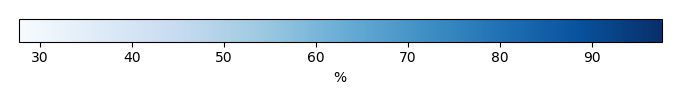MODEL MEANBIAS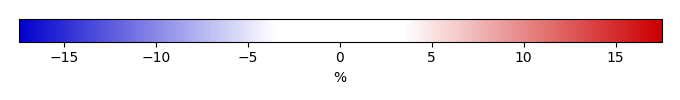BIAS SCORERMSE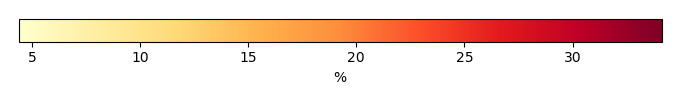RMSE SCOREBENCHMARK INTERANNUAL VARIABILITY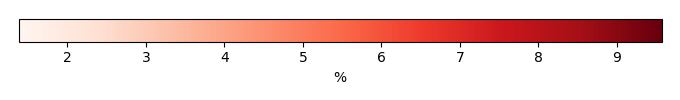MODEL INTERANNUAL VARIABILITYINTERANNUAL VARIABILITY SCOREBENCHMARK MAX MONTHMODEL MAX MONTHDIFFERENCE IN MAX MONTH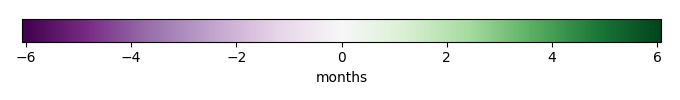SEASONAL CYCLE SCORESPATIAL TAYLOR DIAGRAMMODEL COLORS# Spatially integrated regional mean

MODEL COLORSREGIONAL MEANANNUAL CYCLEMONTHLY ANOMALYANNUAL CYCLE# All Models

BenchmarkCLM5PHSOFFCLM5PHSON# Data Information

creation_date: Wed Dec 2 12:03:21 PST 2015

source_file: This product is generated from monthly ERA interim observations

title: derived ERA interim

Approach: I read the monthly 2m dewpoint temperature and 2m surface air temperature from original file, and calculated 2m relative humidity using the way by John Dutton (Ceaseless Wind, John Dutton. McGraw-Hill, 1976. pp 273-274). The final data were saved by each month and each year.

Temporal resolution: monthly

General information: This product was derived from ERA interim Dataset (1979-present).

Spatial resolution: 0.5x0.5, 1x1 and 2.5x2.5 degree